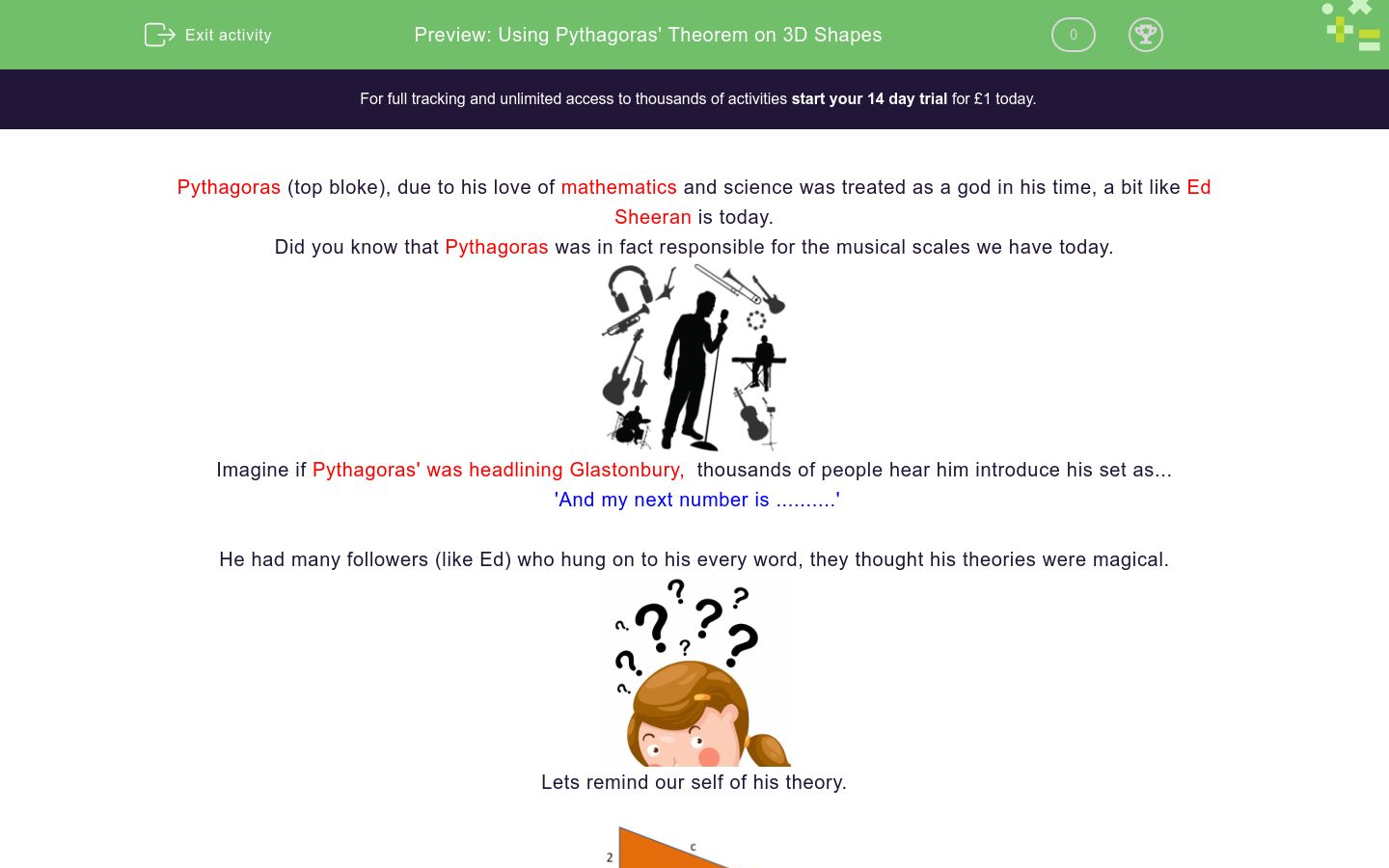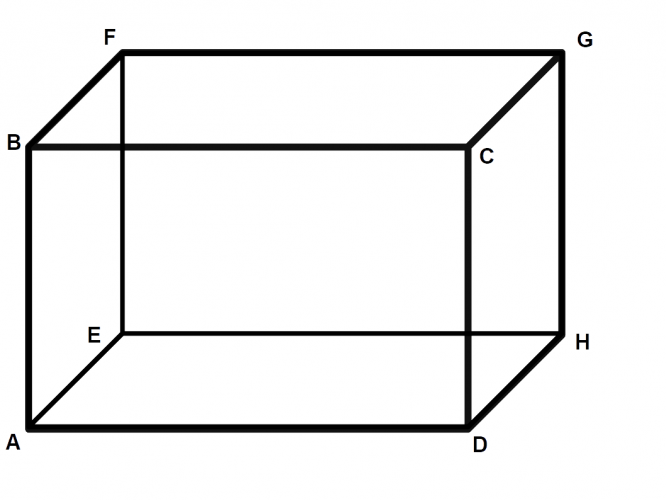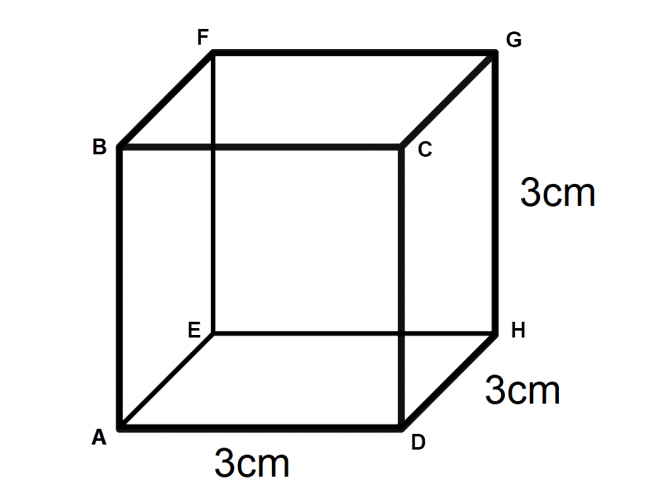# Using Pythagoras' Theorem on 3D Shapes

In this worksheet, students will revise Pythagoras' Theorem and learn how to use it in 3D shapes.Key stage:  KS 4

GCSE Subjects:   Maths

GCSE Boards:   AQA, Eduqas, Pearson Edexcel, OCR,

Curriculum topic:   Geometry and Measures, Mensuration

Curriculum subtopic:   Mensuration and Calculation Triangle Mensuration

Difficulty level:#### Worksheet Overview

In this activity, we will look at using Pythagoras' theorem on 3D shapes.

Let's remind ourselves of the theory with an example:

a² + b² = c²To find the hypotenuse of a triangle:

1. Square one side: 2² = 4²

2. Square the other side: 6² = 36²

4. Square root your answer: √40 = 6.32 (to 2 decimal places)

Pythagoras in 3D

Now let's consider using this in 3D shapes instead of 2D.

Calculating a length is just the same as when working with a 2D triangle; the trick is being able to spot a right-angled triangle within the shape.

Let's look at this cuboid as an example:We can find any diagonal line across any face of a cube or cuboid, as the diagonal forms the hypotenuse of a right-angled triangle

For example:Triangle ADH is a right-angled triangle, and we can use a² + b² = c² to calculate length AH (the hypotenuse).

Here is an overhead view of the base with our line drawn:We can then take this into 3 dimensions by drawing another diagonal through the shape:Here is a cross-section of the shape we have created.We now have a new triangle, AGH, which extends from the bottom corner of the cuboid into the opposite top corner. Significantly, it is still a right-angled triangle, so we can continue to use a² + b² = c² to calculate the hypotenuse, AG. By doing this, we are using Pythagoras in 3D.

Example

Calculate length AG in this cube.The first thing we can do is work out the diagonal across the base, AH:

a2 + b2 = c2

32 + 32 = AH2

AH2 = 18

AH = √18

As we will soon be squaring this answer, it is useful to leave the number in root form at this stage, rather than working with a decimal.

We can then use this length to work out the hypotenuse of triangle AGH:

a2 + b2 = c2

(√18)2 + 32 = AG

AG2 = 18 + 9 = 27

AG = √27 = 5.2 (1 d.p.)

Now that you have seen these examples, have a go at 10 questions to see if you can apply Pythagoras to 3D shapes.

### What is EdPlace?

We're your National Curriculum aligned online education content provider helping each child succeed in English, maths and science from year 1 to GCSE. With an EdPlace account you’ll be able to track and measure progress, helping each child achieve their best. We build confidence and attainment by personalising each child’s learning at a level that suits them.

Get started